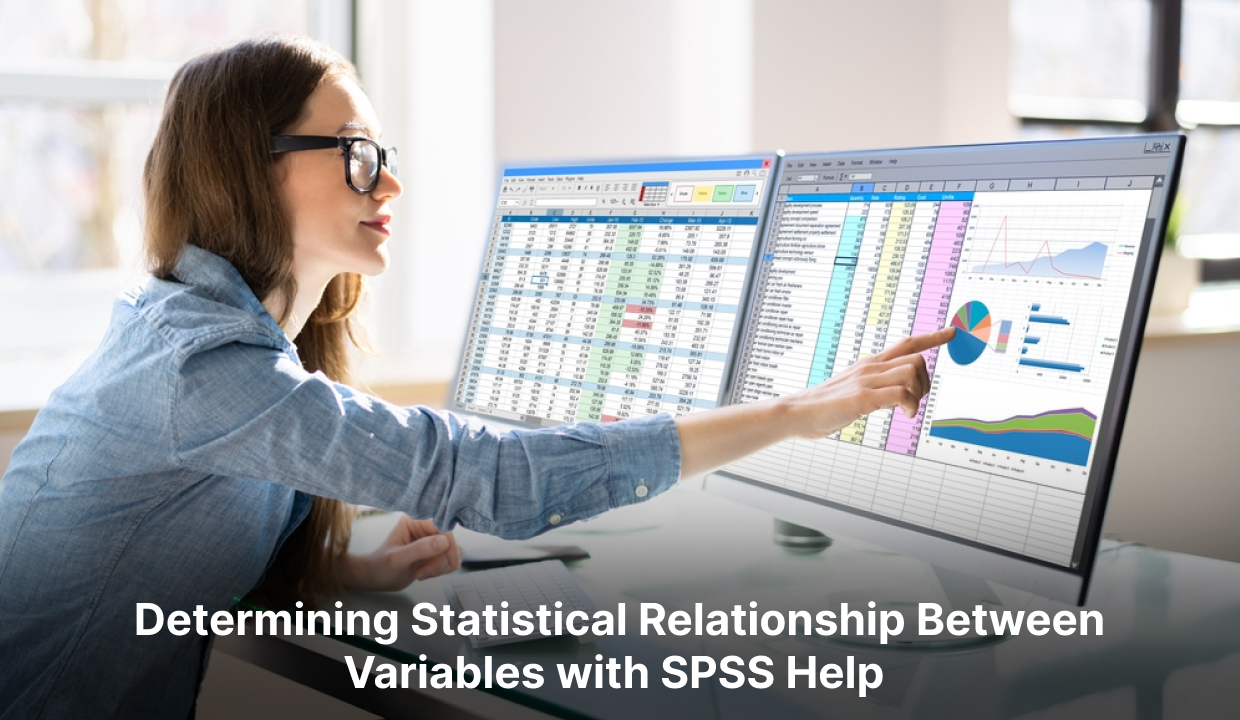# Pearsons Correlation Coefficient Determining Statistical Relationship Between Variables## Introduction

A statistical analysis technique called correlation can be used to evaluate any potential linear relationship between two continuous variables. It is both easy to calculate and explain. However, misuse of correlation is so common among researchers that some statisticians wish the method had never been devised. The purpose of this article is to provide a guide to the proper use of correlation in research. How strongly two variables are related to one another is determined by the correlation coefficient. The most common correlation coefficient is Pearson's, though other varieties exist. Pearson's correlation also known as Pearson's r is a correlation coefficient commonly used in linear regression.

## What is Pearson's correlation coefficient?

The Pearson correlation coefficient, or the Pearson R statistical test, measures the strength of various variables and their relationships. Therefore, it is always a good idea for the person analysing to calculate the value of the correlation coefficient to get a sense of how strong the association is between the two variables whenever any statistical test is performed between two variables.
Pearson's correlation coefficient can range from the value +1 to the value -1, where +1 indicates a perfect positive correlation between the variables considered, -1 indicates a perfect negative correlation between the variables considered, and A value of 0 indicates no relationship between the variables considered.

## Explanation of Pearson's Correlation Coefficient

The Pearson correlation coefficient represents the relationship between two variables, measured on the same interval or ratio scale. It gauges how strongly two continuous variables are correlated. The coefficient not only tells the presence or absence of correlation between two variables but also determines the extent to which those variables are correlated. It is independent of the unit of measurement of the variable where the value of the correlation coefficient can range from the value +1 to the value -1. However, it is insufficient to tell the difference between dependent and independent variables.
It is independent of the unit of measurement of the variables. For example, suppose the unit of measurement of one variable is in years while the unit of measurement of another variable is in kilograms. In that case, this coefficient's value does not change. The correlation coefficient between the variables is symmetric, which means that the correlation coefficient between Y and X or X and Y will have the same value.

## How to determine the statistical relationship between variables

A Pearson's correlation coefficient measures a linear relationship between two variables by determining the strength and direction of its relationship. It is denoted by the symbol "r" and ranges from -1 to +1.
Whenever the correlation coefficient approaches +1, there is a strong positive relationship, meaning that as one variable increases, the other variable also increases. Conversely, a correlation coefficient close to -1 represents a strong negative relationship, indicating that as one variable increases, the other variable decreases. There is no linear relationship between the variables, as indicated by a correlation coefficient of 0.
To calculate Pearson's correlation coefficient, divide the standard deviations of the variables by their covariance. The formula is as follows:

ρ(x,y) = Σ(xi – x̄)(yi – ȳ) / σx*σy
Where:

1. x and y are the values of the variables
2. x̄ and ȳ are the means of x and y, respectively
3. σx and σy are the standard deviations of x and y, respectively

Researchers use correlation analysis & coefficients to determine the strength and direction of a relationship between two variables. Data is often examined and predictions are made by using it in a variety of fields, including statistics, economics, psychology, and social sciences. It is important to keep in mind that correlation does not imply causation. In other words, even a strong correlation between two variables does not demonstrate that one variable caused the change in the other.

## Finding Pearson Correlation Coefficients in SPSS

You can use SPSS to perform the following steps to calculate Pearson correlation coefficients:

1. Launch SPSS and import your data.
2. Go to "Analyse" in the menu bar, click "Correlate," then click "Bivariate."
3. Choose the variables for which you want to compute the Pearson correlation coefficient in the "Bivariate Correlations" dialogue box. The variable names can be moved to the "Variables" box by double-clicking or arrow buttons.
4. Choose "Pearson" as the coefficient to be calculated under the "Correlation Coefficients" section.
5. The "Significant Flag correlations" selection allows you to draw attention to statistically significant correlations.
6. The analysis will begin after you click the "OK" button.
7. The output will appear in the "Output Viewer" window, thanks to SPSS. The Pearson correlation coefficients between the chosen variables are shown in the "Correlations" table.
8. Between -1 and +1 is the Pearson correlation coefficient. Several +1 denotes a perfect linear relationship between the variables, -1 denotes a perfect linear relationship between the variables, and 0 denotes no linear relationship.
9. In SPSS, you may locate Pearson correlation coefficients in the same way.

##Conclusion

The statistical metric is known as Pearson's correlation coefficient, abbreviated "r," assesses the degree and direction of a linear relationship between two continuous variables. It can range from -1 to +1, with +1 being an ideal positive correlation, -1 signifying an ideal negative correlation, and 0 signifying no link. The coefficient is symmetrical between the variables and is independent of the unit of measurement. Researchers can determine the correlation coefficient by using the formula and taking into account the means and standard deviations of the variables. Remembering that a connection does not necessarily indicate a cause and effect is crucial. Software programs like SPSS can facilitate through SPSS help the process of obtaining Pearson correlation coefficients in research. Understanding and interpreting these coefficients can help researchers gain a deeper understanding of the relationships among variables in a wide range of disciplines. Good luck with your research!